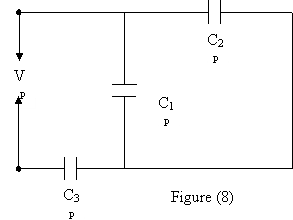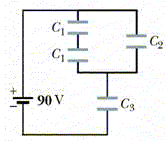KFUPM-Physics102-032
Dr.Aljalal
Sec07
Sec08
Sec09

Recitation ch 26
Answers at the end

Question 1
Two capacitors, C1 and C2, are connected in series and a
potential difference is applied to the combination. If the
capacitor that is equivalent to the combination has the same
potential difference, then the charge on the equivalent
capacitors is the same as:
a   The ratio of the charges on C1 and C2.
b   The sum of the charges on C1 and C2.
c   The charge on C1 or C2.
d   The difference of the charges on C1 and C2.
e   The product of the charges on C1 and C2.
==============================================
Question 2
Capacitor C1 has a capacitance of 4 micro-Farad.
It is charged using a 100-volt battery. The battery
is disconnected. Then, C1 is connected in parallel
to another uncharged capacitor C2. Now, the potential
difference between the plates of each capacitor is 40 V.
What is the value of C2 ?

==============================================
Question 3
In figure (8), find the total charge stored by the three
capacitors if the potential difference ōVö is 10.0 volts.
Assume C1 = 10.0 micro-F, C2 = 5.00 micro-F and
C3 = 4.00 micro-F.a   26.1 micro-C.
b   61.3 micro-C.
c   22.1 micro-C.
d   31.6 micro-C.
e   63.4 micro-C.
==============================================
Question 4
Capacitors A and B are identical. Capacitor A is charged so
it stores 4 J of energy and capacitor B is uncharged. The
capacitors are then connected in parallel. The total stored
energy in the capacitors is now:

a   16 Joules.
b   2 Joules.
c   4 Joules.
d   1 Joules.
e   8 Joules.
==============================================
Question 5
In figure 6: V = 100 V, C1 = 10.0, C2 = 5.00, C3 = 4.00.
All capacitor values are in microFarads.
What is the energy stored in C1 ?a   50.0 mJ
b   5.56 mJ
c   1.12 mJ
d   11.2 mJ
e   10.3 mJ
==============================================
Question 6
The space between the plates of a 2.0-nanoFarad parallel-plate
capacitor is completely filled with a dielectric of dielectric
constant k = 5. A battery is used to charge the capacitor to a
potential difference of 100 V. Then the battery is disconnected
and the dielectric is pulled completely out of the capacitor.
What is the final energy stored in the capacitor ?

a   1.0*10**(-5) J
b   5.0*10**(-5) J
c   0.2*10**(-5) J
d   0.5*10**(-5) J
e   2.0*10**(-5) J
==============================================
Question 7
A 2 micro-F and a 1 micro-F capacitor are connected in series
and a potential difference is applied across the combination.
The 2 micro-F capacitor has:

a  half the charge of the 1 micro-F capacitor.
b  zero of stored energy.
c  twice the potential difference of the 1 micro-F capacitor.
d  twice the charge of the 1 micro-F capacitor.
e  half the potential difference of the 1 micro-F capacitor.
==============================================
Question 8
A parallel-plate capacitor has a plate area of 0.2 m**2 and
a plate separation of 0.1 mm. If the charge on each plate has
a magnitude of 4.0*10**(-6) C the electric field between the
plates is approximately:

a   Zero.
b   1.4*10**4 V/m.
c   9.2*10**3 V/m.
d   2.3*10**6 V/m.
e   4.2*10**6 V/m.
==============================================
Question 9
A parallel plate capacitor has a plate area of 0.2 m**2
and a plate separation of 0.1 mm. The charge on each
plate is 4.0 microCoulombs.
What is the electric field between the plates ?

a   1.0*10**6 V/m
b   zero
c   4.0*10**2 V/m
d   2.3*10**6 V/m
e   4.0*10**12 V/m
==============================================
Question 10
In figure 7, C1 = 12 micro-Farads, C2 = 6 micro-Farads
and C3 = 20 micro-Farads.
What is the total energy stored by the capacitors ?a   88.4 mJ
b   0.981 mJ
c   0.100 mJ
d   30.4 mJ
e   0.491 mJ
==============================================
Question 11
An air filled parallel-plate capacitor has a capacitance of
1.00*10**(-12) F. The plate separation is then doubled and a
wax dielectric is inserted, completely filling the space
between the plates. As a result the, capacitance becomes
2.00*10**(-12) F. The dielectric constant of the wax is:

a   4.00.
b   8.00.
c   0.25.
d   2.00.
e   0.50.
==============================================
Question 12
Find the equivalent capacitance of three capacitors
connected in series. Assume the three capacitors are:
C1 = 2.00 micro-F, C2 = 4.00 micro-F and
C3 = 8.00 micro-F.

a   15.4 micro-F.
b   26.1 micro-F.
c   3.01 micro-F.
d   1.14 micro-F.
e   0.88 micro-F.
==============================================
Question 13
Figure 5 shows six capacitors each having a capacitance
The capacitance between points a and b is:==============================================
Question 14
A battery is used to charge a parallel-plate capacitor.
The battery is disconnected, and then, the plates are
pulled apart to twice their original separation.
This process will double the :

a   surface charge density on each plate
b   electric field between the plates
c   capacitance
d   stored energy
e   charge on each plate
==============================================
Question 15
The potential difference between the plates of an
isolated, charged capacitor is Vo. A slab of dielectric
material is inserted filling completely the space between
the plates. The potential difference across the capacitor
is now Vo/2. Find the dielectric constant of the material.

a   0.5
b   16
c   2
d   8
e   4
==============================================

1     c
2     d
3     d
4     b
5     b
6     b
7     e
8     d
9     d
10     d
11     a
12     d
13     d
14     d
15     c